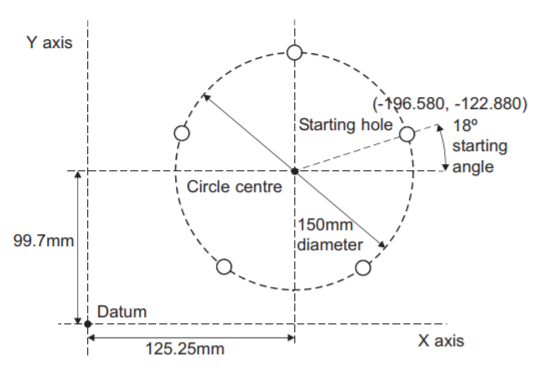The DP700 calculates positions for a series of equally spaced holes around the circumference of a circle. The message display prompts the user for various parameters it needs to do the calculations.

Once the DP700 completes the calculations, the axis displays show the distance to each hole. The operator works to Zero for each hole location. See example below.

## How to navigate to PCD function.## Using Set Mode

With set mode selected, this enables the select axis keys to prompt a numeric entry into the desired axis. Once the correct value has been selected, it can be set into the axis by pressing the enter key. This can be seen in the example on the right.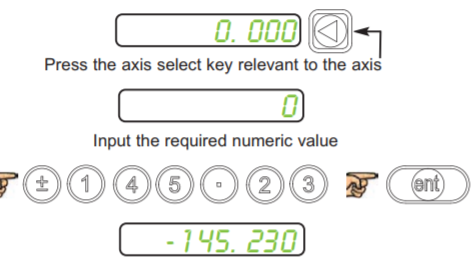## Example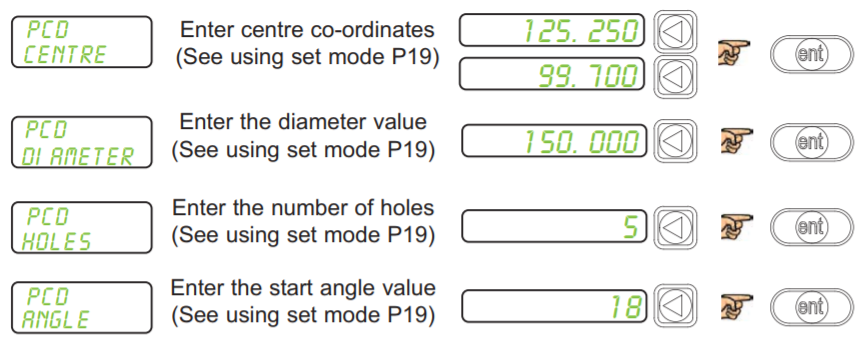Note: The PCD will be calculated from the 3 0‘clock position, anti-clockwise. Enter the angle as a negative value if it is given as clockwise from 3 o’clock.

Note: At this point you can use the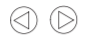keys to navigate back and forth through the above menus.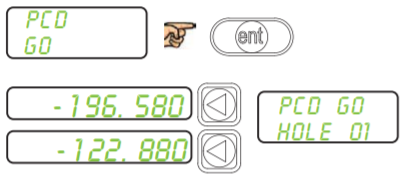Note: The numbers appear as negative values because the operator works to zero

Navigate through the sequence of holes by usingkeys

The maximum number of holes is 999

## Bolt Hole Function Diagram